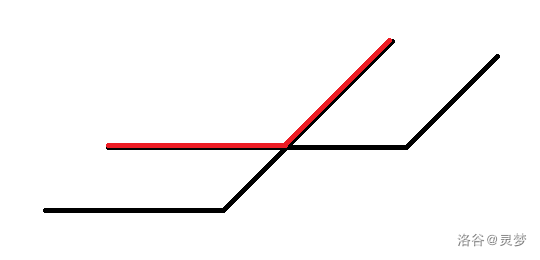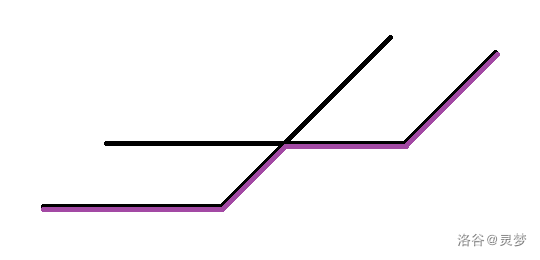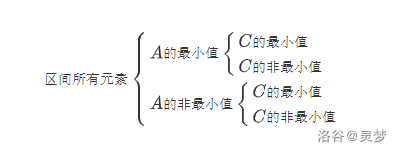# 灵梦 的博客# 灵梦 的博客

### 区间最值操作与区间历史最值详解

posted on 2020-03-20 17:44:20 | under 未分类 |

# 区间最值操作

#### HDU5306. Gorgeous Sequence

1. 给出 $l,r,k$ ，对于所有 $i\in[l,r]$ ，将 $A_i$ 变成 $\min(A_i,k)$ ；
2. 给出 $l,r$ ，求序列 $A$ 区间 $[l,r]$ 的最大值；
3. 给出 $l,r$ ，求 $\sum_{i=l}^{r}A_i$ 。

• $k\geq mx$ ，此时该操作不会对当前节点产生影响，直接退出；
• $se<k<mx$ ，此时这个节点维护的区间中所有最大值都会被修改为 $k$ ，而最大值个数不变。将区间和加上 $cnt\cdot(k-mx)$ ，打上懒标记，然后退出即可；
• $k\leq se$ ，此时无法快速更新区间信息，因此我们需要继续递归到左右子树中，回溯时合并信息。void update(int o, int k) // 只打标记不下传
{
if (k<tr[o].mx)
// 这里需要判一下当前节点是否为最大值
{
tr[o].sum+=(1ll*k-tr[o].mx)*tr[o].cnt;
tr[o].mx=tr[o].tag=k;
}
}

void pushdown(int o) // 下传标记到左右子树
{
if (~tr[o].tag)
{
update(lc, tr[o].tag);
update(rc, tr[o].tag);
tr[o].tag=-1; // 这个题里没有负数，把标记清为 -1 即可
}
}

void modify(int o, int l, int r, int k)
{
if (tr[o].l>r||tr[o].r<l||k>=tr[o].mx) return; // 情况 1
if (l<=tr[o].l&&tr[o].r<=r&&k>tr[o].se)
{ update(o, k); return; } // 情况 2
pushdown(o);
modify(lc, l, r, k), modify(rc, l, r, k); // 继续在子树内搜索
pushup(o);
}

## 支持区间加减

• 套用上一题的做法，我们只需把标记换成二元组 $(add, v)$ ，表示将当前节点维护的区间先加上 $add$ 再与 $v$ 取最小值。合并标记时，先考虑给当前节点加上一个标记，我们除了更新当前节点的区间加标记 $add$ ，还需要把它加到 $v$ 上；而给当前节点取最小值可以直接将标记对它取 $\min$ 。形式地说，当节点 $x$ 下传标记到它的儿子 $ch$ 时，我们把儿子的标记更新为 $(add_{ch}+add_x,\min(v_{ch}+add_x,v_x))$ 即可。
• 换一种新的思路，我们发现这种方法的本质是把线段树上每个节点维护的元素分成了最大值和非最大值这两类，并对它们分别维护。区间最大值标记只会打在 $se<v<mx$ 的节点上，可以看成只针对最大值的加减操作；而区间加操作对于这两类值都生效。分别维护区间最大值的加减标记和非最大值的加减标记，下传时判断当前节点是否为区间最大值即可。

## 同时支持区间最小值和最大值

#### BZOJ4695. 最假女选手

1. 给出 $l,r,k$ ，对于所有 $i\in[l,r]$ ，将 $A_i$ 加上 $k$ ；
2. 给出 $l,r,k$ ，对于所有 $i\in[l,r]$ ，将 $A_i$ 变成 $\max(A_i,k)$ ；
3. 给出 $l,r,k$ ，对于所有 $i\in[l,r]$ ，将 $A_i$ 变成 $\min(A_i,k)$ ；
4. 给出 $l,r$ ，求 $\sum_{i=l}^{r}A_i$ ；
5. 给出 $l,r$ ，求序列 $A$ 区间 $[l,r]$ 的最大值；
6. 给出 $l,r$ ，求序列 $A$ 区间 $[l,r]$ 的最小值。

• 对于加减操作，在线段树上定位区间后直接对三类值同时加上 $k$ ；
• 对于取最小值操作，在线段树上暴力搜索找到 $mx2<k<mx1$ 的节点。这些节点对应的区间的最大值都应该改成 $k$ ，只对最大值加上 $k-mx1$ 即可。
• 取最大值操作同理。

• 以最大值上的加减标记为例。下传这个标记时要判断子区间内是否包含最大值，如果不包含则应下传其他值的加减标记；
• 如果一个区间的值域很小（只有 $1$ 或 $2$ 个数），可能会发生一个值既是最大值又是次小值这种情况，也就是发生了数域的重叠。这种情况要特判，分辨到底该被哪个标记作用。

// add1, add2, add3 分别表示最小值、最大值和其他值的加减标记。
// k1, k2, k3 也是按这个顺序。
void update(int o, int k1, int k2, int k3)
// 作用标记并合并标记，一定要注意顺序
{
if (tr[o].mn1==tr[o].mx1)// 只有一种值时，最大值等于最小值
{
if (k1==k3) k1=k2; else k2=k1; // 不应被其他值的标记作用
tr[o].sum+=1ll*k1*tr[o].cmn;
}
else tr[o].sum+=1ll*k1*tr[o].cmn+1ll*k2*tr[o].cmx
+1ll*k3*(tr[o].r-tr[o].l+1-tr[o].cmn-tr[o].cmx);

if (tr[o].mn2==tr[o].mx1) tr[o].mn2+=k2;
// 次小值等于最大值，应该被最大值标记作用
else if (tr[o].mn2!=INF) tr[o].mn2+=k3;
// 否则应该被其他值标记作用

if (tr[o].mx2==tr[o].mn1) tr[o].mx2+=k1;
else if (tr[o].mx2!=-INF) tr[o].mx2+=k3;
// 对次大值同理

tr[o].mn1+=k1, tr[o].mx1+=k2;
}

void pushdown(int o) // 下传标记
{
int mn=min(tr[lc].mn1, tr[rc].mn1);
int mx=max(tr[lc].mx1, tr[rc].mx1);
// 找出最大值和最小值

// 下传标记到左子树，若左子树中有最大值则下传最大值加减标记，否则下传其他值加减标记。对最小值同理
// 右子树同理

}

## 一个应用的例子

#### UOJ#515. 【UR #19】前进四

1. 给出 $x,v$ ，将 $A_x$ 变成 $v$ ；
2. 给出 $x$ ，求 $A_x,A_{x+1},\cdots,A_n$ 的不同后缀最小值个数。

# 区间历史最值

## 沿用懒标记

#### BZOJ3064. Tyvj 1518 CPU监控

1. 给出 $l,r,k$ ，对于所有 $i\in[l,r]$ ，将 $A_i$ 加上 $k$ ；
2. 给出 $l,r,k$ ，对于所有 $i\in[l,r]$ ，将 $A_i$ 变成 $k$ ；
3. 给出 $l,r$ ，求序列 $A$ 区间 $[l,r]$ 的最大值；
4. 给出 $l,r$ ，求序列 $B$ 区间 $[l,r]$ 的最大值。

// 以下代码段中，所有带下划线的变量都是历史最值
void plus(int o, int k, int k_) // 区间加标记
{
tr[o].mx_=max(tr[o].mx_, tr[o].mx+k_), tr[o].mx+=k;
// 更新历史最大值和当前最大值，这里要注意顺序
if (!tr[o].tag)
else tr[o].cov_=max(tr[o].cov_, tr[o].cov+k_), tr[o].cov+=k;
// 否则直接加到 cov 上
}

void cover(int o, int k, int k_) // 区间覆盖标记
{
tr[o].mx_=max(tr[o].mx_, k_), tr[o].mx=k;
tr[o].cov_=max(tr[o].cov_, k_), tr[o].cov=k, tr[o].tag=1;
}

void pushdown(int o) // 下传标记
{
{
}
if (tr[o].tag) // 这里的 tag 表示当前节点有没有区间覆盖标记
{
cover(lc, tr[o].cov, tr[o].cov_);
cover(rc, tr[o].cov, tr[o].cov_);
tr[o].tag=0, tr[o].cov_=INT_MIN;
}
}

## 与区间最值操作结合

### 区间最值操作与历史最值询问同向

#### UOJ#164. 【清华集训2015】V

1. 给出 $l,r,k$ ，对于所有 $i\in[l,r]$ ，将 $A_i$ 加上 $k$ ；
2. 给出 $l,r,k$ ，对于所有 $i\in[l,r]$ ，将 $A_i$ 变成 $\max(A_i-k,0)$ ；
3. 给出 $l,r,k$ ，对于所有 $i\in[l,r]$ ，将 $A_i$ 变成 $k$ ；
4. 给出 $p$ ，询问 $A_p$ ；
5. 给出 $p$ ，询问 $B_p$ 。### 区间最值操作与历史最值询问反向

#### UOJ#169. 【UR #11】元旦老人与数列

1. 给出 $l,r,k$ ，对于所有 $i\in[l,r]$ ，将 $A_i$ 加上 $k$ ；
2. 给出 $l,r,k$ ，对于所有 $i\in[l,r]$ ，将 $A_i$ 变成 $\max(A_i,k)$ ；
3. 给出 $l,r$ ，求序列 $A$ 区间 $[l,r]$ 的最小值；
4. 给出 $l,r$ ，求序列 $B$ 区间 $[l,r]$ 的最小值。• 最小值加减标记
• 最小值历史最小的加减标记
• 非最小值加减标记
• 非最小值历史最小的加减标记

## 维护历史最值和

1. 给出 $l,r,k$ ，对于所有 $i\in[l,r]$ ，将 $A_i$ 加上 $k$ ；
2. 给出 $l,r$ ，求 $\sum_{i=l}^{r}B_i$ 。

• $A_i+k\leq B_i$ 。此时 $B_i$ 不会发生变化，所以 $C_i\gets C_i+k$ ；
• $A_i+k>B_i$ 。此时 $B_i$ 也会变成 $A_i+k$ ，所以 $C_i\gets 0$ 。